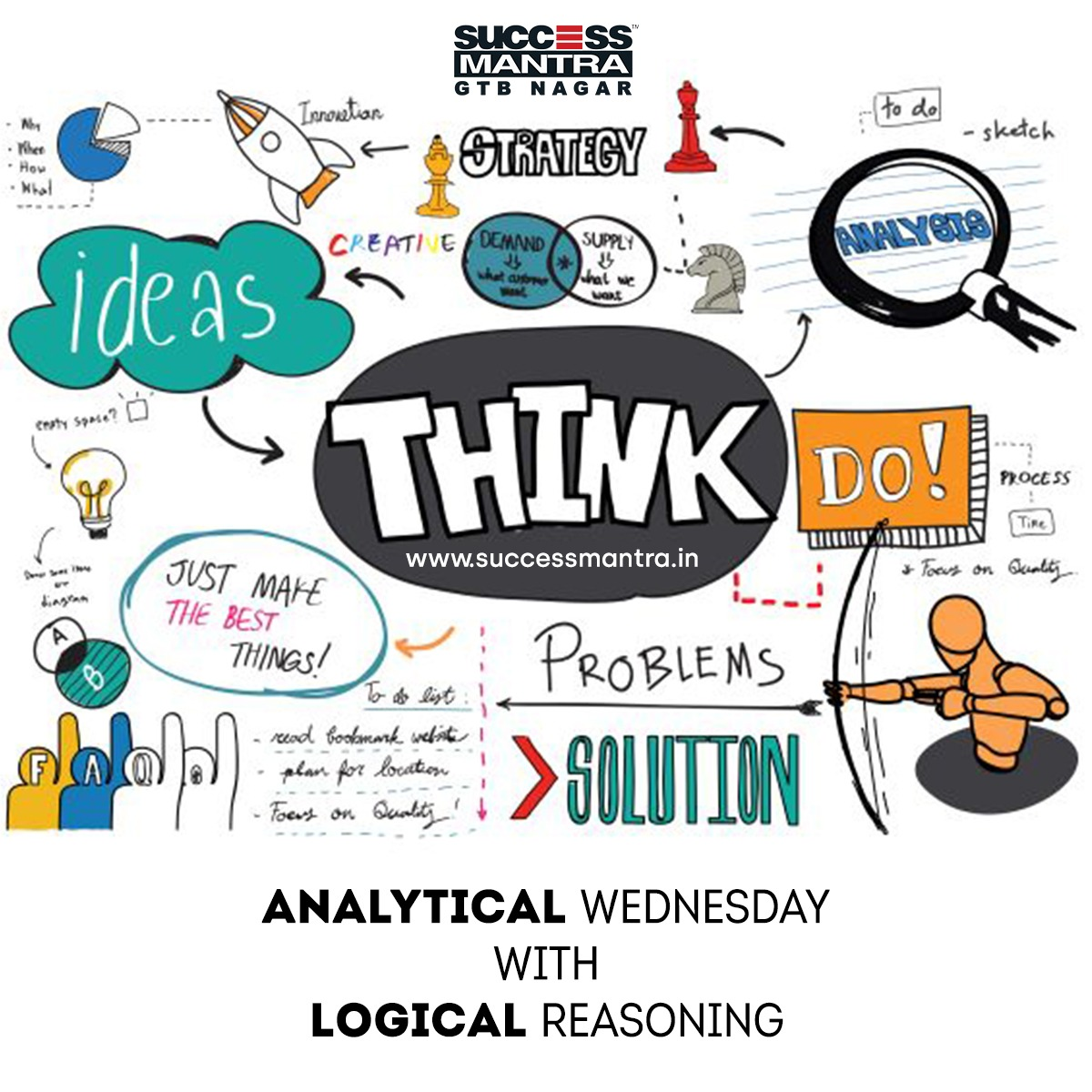## Questions on Logical Reasoning SMLRQ030Directions (1-5): Study the following information carefully and answer the questions given below:

In a certain code language,
‘IBPS is agency’ is written as ‘N D G’
‘PO occupy manager’ is written as ‘E J B’
‘HR are clerk’ is written as ‘H O C’
‘Banks are economy’ is written as ‘H F K’
‘Manager takes HR’ is written as ‘O J M’
‘RBI funds country’ is written as ‘L I A’
‘Country occupy banks’ is written as ‘L F E’
‘Economy is RBI’ is written as ‘K I N’
‘Economy takes agency’ is written as ‘G M K’
After coding the words, fill the following matrix accordingly and answer the questions given below:

 RBI Manager Are HR funds IBPS Clerk Is Banks Agency PO Economy Takes Occupy country

1. Which alphabet is exactly between D and N in the matrix?

(a) K
(d) F
(c) H
(d) B

2. Which alphabet is immediate right above diagonally of E?

(a) N
(b) G
(c) K
(d) I

3. Which alphabet is third to the right of J in the matrix?

(a) G
(b) G
(c) K
(d) L

4. Which alphabet is immediate left below diagonally of O?

(a) N
(b) G
(c) C
(d) M

5. Which alphabet is second to the left of M in the matrix?

(a) I
(b) C
(c) J
(d) B